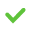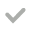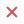# Type Conversion Rules

Most expressions or functions in SQL expect a particular data type, for example, a CHAR or VARCHAR data type in a string function SUBSTR[ING]. If you wish to use a different data type, it must be converted first using the explicit conversion functions. For more information, see Conversion Functions.

Data types can be converted either explicitly or implicitly. If explicit conversion functions are not used, then the system attempts to perform an implicit conversion. If an implicit function is not possible or if one single value is not successfully converted during the computation, the system displays an error message.

The following table provides you with an overview of the permissible implicit conversions.

SOURCE TYPE
TARGET TYPE
DECIMAL
DOUBLE
BOOLEAN
DATE/TIMESTAMP
INTERVAL YEAR[(p)] TO MONTH
INTERVAL DAY[(p)] TO SECOND[(fp)]
GEOMETRY
HASHTYPE
CHAR/VARCHAR

DECIMALDOUBLEBOOLEANDATE/TIMESTAMPINTERVAL YEAR[(p)] TO MONTHINTERVAL DAY [(p)] TO SECOND[(fp)]GEOMETRYHASHTYPECHAR/VARCHAR= Implicit conversion always works= Implicit conversion possible, but errors may occur if single values cannot be converted= Implicit conversion is not possible

Implicit conversions between `HASHTYPE` and `CHAR/VARCHAR` data types are not supported in joins in order to maintain the performance improvements of using the `HASHTYPE` data type.

## Conversion Considerations

Below are some of the conversion behaviors exhibited by their corresponding data types:

• When converting DECIMAL to DOUBLE, inaccuracies may occur with rounding.
• When converting a DECIMAL to a DECIMAL with a lower scale (including the INTEGER alias), the value is rounded to the scale specified. For example, SELECT CAST(5.56 AS DECIMAL(3,1)); will return 5.6.
• When converting DECIMAL or DOUBLE to BOOLEAN, 1 is transformed to TRUE, 0 is transformed to FALSE, and NULL is transformed to NULL. For other data types, you can use the compatible representations of the values 1 and 0. For example, 1 = 1.00 (DECIMAL(3.2)) = 1.0 (DECIMAL(2.1)).
• When converting from BOOLEAN to DECIMAL or DOUBLE, TRUE is transformed to 1, FALSE is transformed to 0, and NULL is transformed to NULL.
• When converting from BOOLEAN to CHAR(n) or VARCHAR(n), TRUE is transformed to 'TRUE', and FALSE is transformed to 'FALSE'.
• The conversion of GEOMETRY object with different spacial reference systems is not supported.
• The conversion from CHAR(n) or VARCHAR(n) functions if n is large enough and all characters exist in the target character set.
• The conversion from CHAR(n) or VARCHAR(n) to another data type is successful only if the data to be converted conforms to the target data type.
• When converting from CHAR(n) or VARCHAR(n) to DATE or TIMESTAMP, you must take into account the current format model. For more information , see Date/Time Format Model section.
• When converting from CHAR(n) or VARCHAR(n) to BOOLEAN, you can use strings '0', 'F', 'f' 'N' 'n' or 'FALSE' (case-insensitive) for value FALSE and strings '1', 'T', 't' 'y' 'Y' or 'TRUE' (case-insensitive) for value TRUE.
• When there are operations with multiple operands (for example, the operators +,-,/,*), Exasol implicitly converts the operand to the data type with higher precedence (for example, DOUBLE is higher than DECIMAL) before executing the operation. This rule is also called numeric precedence.
• When converting between GEOMETRY types and VARCHAR/CHAR data types, the maximum significant digits used when casting is defined with the ST_MAX_DECIMAL_DIGITS parameter and can be changed using the ALTER SYSTEM or ALTER SESSION statements. Note that the default value is 16, and the allowed range is <1,16>.
• For example, the table for POINT(40.7267, -74.0345) is casted to string with different precisions, as shown below:

ST_MAX_DECIMAL_DIGITSGeometry as a string
1POINT(4e+01 -7e+01)
2POINT(41 -74)
3POINT(40.7 -74)
4POINT(40.73 -74.03)
5POINT(40.727 -74.034)
6 POINT(40.7267 -74.0345)
7 POINT(40.7267 -74.0345)
8 POINT(40.7267 -74.0345)
9 POINT(40.7267 -74.0345)
10 POINT(40.7267 -74.0345)
11 POINT(40.7267 -74.0345)
12 POINT(40.7267 -74.0345)
13 POINT(40.7267 -74.0345)
14 POINT(40.7267 -74.0345)
15 POINT(40.7267 -74.0345)
16POINT(40.7267 -74.03449999999999)

###### Example

In the following example, implicit conversion is conducted to insert the BOOLEAN entry into the DECIMAL column of the created table.

``CREATE TABLE t(d DECIMAL);-- Implicit conversion from BOOLEAN to DECIMALINSERT INTO t VALUES TRUE, NULL, FALSE;SELECT * FROM t;``